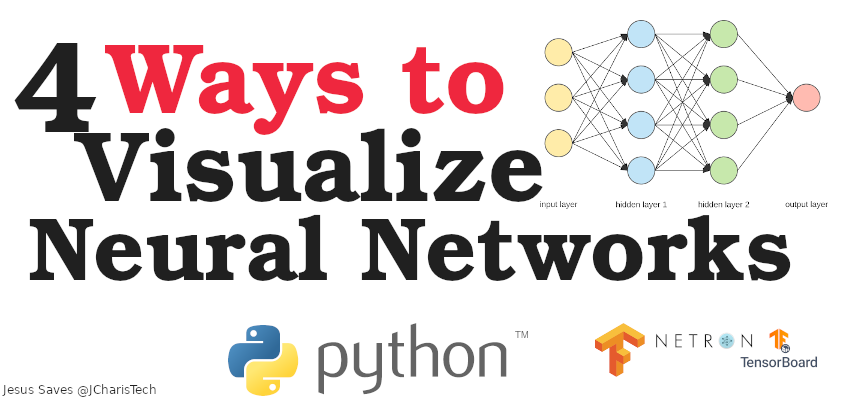# How to Visualize Neural Networks

Neural Networks or preferably called Artificial neural networks forms the building block for Deep learning activities.

In this article, we will explore the various ways we can visualize your neural network architecture. We will be using 4 different methods to help us visualize the components of a neural network. By the end of this tutorial we will learn about

• tools to visualize neural networks
• how to visualize your neural networks
• how to use tensorboard
• etc

### Tools to Visualize Neural Networks

There are several tools and package that we can use to visualize neural networks. In this tutorial we will talk about 4 of them. These tools or packages include

• Plot_model from TensorFlow
• ANN-Visualizer
• Netron
• Tensorboard

#### Tensorflow/Keras Plot_model

Keras/Tensorflow comes with a native function to help visualize the components and the structure of your artificial neural network. The plot_model() function can be used to visualize any keras-related or tensorflow generated neural network. This will give you a flow chart of the input, the layers and the output for your artificial neural network. The plot_model function takes as input the model and then the filename you want to save your plot as via the ‘to_file‘ argument

``````# Load utils
from tensorflow.keras.utils import plot_model

model = Sequential()
.....

# Visualize the model
plot_model(model,to_file='my_ann_model.png',show_shapes=False)

# Visualize the Model showing the input and output shapes
plot_model(model,to_file='my_ann_model.png',show_shapes=True)``````

#### ANN-Visualizer

This is another alternative to visualizing the component of a neural network, however there can be some challenges when using this tool. Howbeit it is quite simple to use.

To install it you can use pip via

```pip install ann-visualizer
```

In order to use Ann-Visualizer you can do the following

``````from ann_visualizer.visualize import ann_viz
import graphviz

# Usage
ann_viz(model,filename='my_ann_model.gv',title='Artificial Neuron')
graph_file = graphviz.Source.from_file('my_ann_model.gv')
graph_file``````

#### Netron

Netron is one of the alternative. It comes as a standard alone desktop software that is cross-platform compatible since it was built with electron and react. Moreover there is also a free online service by the same team that made netron for visualizing the components of an ANN.

You can install netron as follows

```# For Python
pip install netron
# For Linux
snap install netron
# For Mac
brew install netron```

In order to use netron, you will have to save your neural network model as h5 or any other format and then upload it to netron app or the online service. That is it

#### Tensorboard

The final but not the least important is tensorfboard from tensorflow. This library has several features beside visualizing the components of a neural network. It offers tons of features.

To utilize it , you will have to install it via pip as below

`pip install tensorboard`

You can also check out the video tutorial below.

Let me know any package you find that we can use to visualize ANN and tell me your favorite amongst these 4.